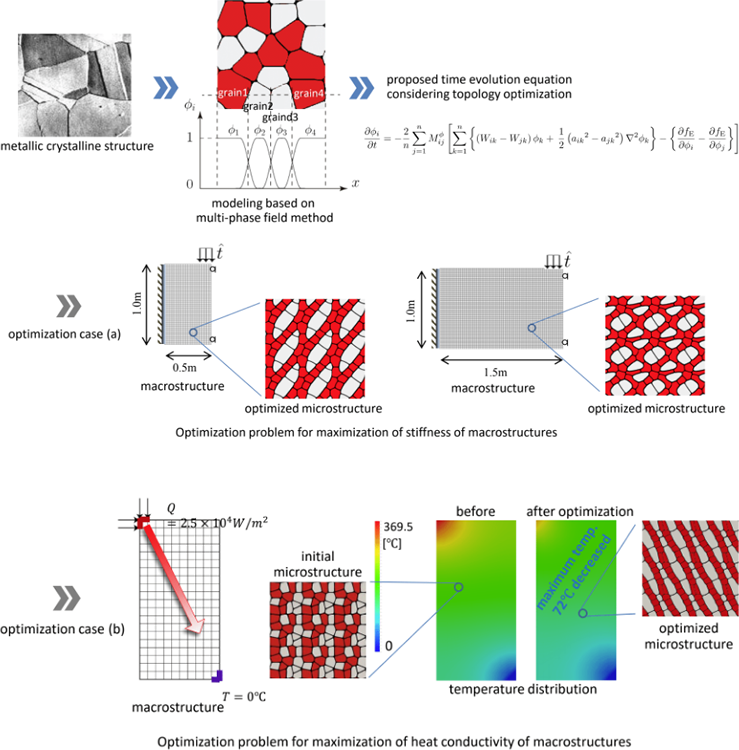top of page

# Meta-material design with negative Poisson’s ratio

Metamaterials are artificial materials that behave in ways that are not found in nature. In this research, we are developing a method for calculating a material microstructure with a negative Poisson's ratio (the behavior of an object that deforms inward in the horizontal direction when compressed in the vertical direction), which is a typical example, using finite element analysis based on the homogenization theory. We are developing a method to calculate the microstructure of materials with a negative Poisson's ratio. Materials with a negative Poisson's ratio are expected to be applied to wire materials that expand in the diameter direction when subjected to tensile deformation. We are also working on the optimal design of materials with a "negative coefficient of thermal expansion" (i.e., behavior that shrinks or does not deform when heat is applied).# Multi-scale topology optimization considering elastoplastic deformation

In this study, we are investigating a multiscale topology optimal design method that takes into account the microscopic elastoplastic behavior that occurs in the microstructure of materials such as Fiber-Reinforced Plastics (FRP). We are developing a method for determining the optimal material configuration in the material microstructure that maximizes the energy absorption performance of the macrostructure within the framework of a decoupled multiscale analysis, assuming von Mises' isotropic hardening elasto-plastic material model in the microdomain and Hill's anisotropic elasto-plastic material model in the macrodomain, with the material volume as a constraint. We are developing a method for determining the optimal material configuration of material microstructures to maximize the energy absorption performance of macrostructures in the framework of separated multiscale analysis.# Micro-macro concurrent topology optimization with kinematical nonlinearity

In this study, we are developing a method to simultaneously optimize both the material microstructure and the macrostructure topology in order to maximize the end compliance of the macrostructure. Here, the microstructure is assumed to consist of two-phase multi-materials, each of which is modeled by Mooney-Rivlin hyperelasticity, and the macrostructure is modeled by Kaliske anisotropic hyperelasticity law to show the optimal structure and material when finite deformation behavior is considered. The left side shows the optimal results for small loads, and the right side shows the optimal results for large loads.# Optimal design for metallic micro-structure applying a multi-phase field-based multi-scale topology optimization

In recent years, the development of a technology that enables modeling of metallic materials with a certain degree of control over the crystal structure, which is the "heart" of the material, has been promoted. In this research, we are trying to find out the microstructure of metal that expresses the desired material properties by computer. The following is an example of the computer calculation of (a) the material microstructure that maximizes the stiffness and (b) the material microstructure that maximizes the heat conduction performance of a macrostructure, which was optimally designed by incorporating the multi-phase field method into the framework of multi-scale analysis.bottom of page